# ICSE Solutions for Selina Concise Chapter 26 Co-ordinate Geometry Class 9 Maths

### Exercise 26(A)

1. For the equation given below; name the dependent and independent variables

(i) y = (4/3)x  - 7

(ii) x = 9y + 4

(iii) x = (5y+3)/2

(iv) y = (1/7)(6x + 5)

(i) y = (4/3)x  - 7
Dependent variable is y
Independent variable is x

(ii) x = 9y + 4
Dependent variable is  x
Independent variable is  y

(iii) x = (5y + 3)/2
Dependent variable is  x
Independent variable is  y

(iv) y = (1/7) (6x + 5)
Dependent variable is  y
Independent variable is  x

2. Plot the following points on the same graph paper:

(i) (8, 7)
(ii) (3, 6)
(iii) (0, 4)
(iv) (0, -4)
(v) (3, -2)
(vi) (-2, 5)
(vii) (-3, 0)
(viii) (5, 0)
(ix) (-4, -3)

Let us take the point as
A(8, 7), B(3, 6), C(0, 4), D(0, -4), E(3, -2), F(-2, 5), G(-3, 0), H(5,0), I(-4, -3)
On the graph paper, let us draw the co-ordinate axes XOX’ and YOY’ intersecting at the origin O. With proper scale, mark the numbers on the two co-ordinate axes.

Now for the point A(8, 7)
Step I: Starting from origin O, move 8 units along the positive direction of X axis, to the right of the origin O
Step II: Now from there, move 7 units up and place a dot at the point reached. Label this point as A(8,7)
Similarly plotting the other points,
B(3,6), C(0,4), D(0, - 4), E(3, - 2), F(-2, 5), G(-3,0), H(5,0), I(-4, -3)

3. Find the values of x and y if:

(i) (x – 1, y + 3) = (4, 4)

(ii) (3x + 1, 2y – 7) = (9, – 9)

(iii) (5x – 3y, y – 3x) = (4, -4)

Two ordered pairs are equal.
⇒ Therefore their first components are equal and their second components too are separately equal.

(i) (x-1, y+3) = (4, 4)
(x-1, y+3) = (4, 4)
⇒ x -1 = 4 and y + 3 = 4
⇒ x =5 and y = 1

(ii) (3x + 1, 2y -7) = (9, -9)
3x +1 = 9 and 2y -7 = -9
⇒ 3x = 8 and 2y = -2
⇒ x = 8/3 and y = -1

(iii) (5x - 3y, y - 3x) = (4, -4)
5x - 3y = 4 ...(A) and  y - 3x = -4 ...(B)
Now multiplying the equation (B) by 3, we get
3y -9x = -12 ...(C)
Now adding both the equations (A) and (C),  we get
(5x - 3y) + (3y - 9x) = ( 4 + (-12))
⇒ - 4x = -8
⇒ x = 2
Putting the value of x in the equation (B), we get
y - 3x = -4
⇒ y = 3x - 4
⇒ y = 3(2) - 4
⇒ y = 2
Therefore we get,
x = 2, y = 2

4 . Use the graph given alongside, to find the coordinates of the point (s) satisfying the given condition:
(i) The abscissa is 2.
(ii)The ordinate is 0.
(iii) The ordinate is 3.
(iv) The ordinate is -4.
(v) The abscissa is 5.
(vi) The abscissa is equal to the ordinate.
(vii) The ordinate is half of the abscissa.

(i) The abscissa is 2
Now using the given graph the co-ordinate of the given point A is given by (2,2)
(ii) The ordinate is 0
Now using the given graph the co-ordinate of the given point B is given by (5,0)
(iii) The ordinate is 3
Now using the given graph the co-ordinate of the given point C and E is given by (-4,3)& (6,3)
(iv) The ordinate is -4
Now using the given graph the co-ordinate of the given point D is given by (2,-4)
(v) The abscissa is 5
Now using the given graph the co-ordinate of the given point H, B and G is given by (5,5) ,(5,0) & (5,-3)
(vi)The abscissa is equal to the ordinate.
Now using the given graph the co-ordinate of the given point I,A & H is given by (4,4),(2,2) & (5,5)
(vii)The ordinate is half of the abscissa
Now using the given graph the co-ordinate of the given point E is given by (6,3)

5. State, true or false:

(i) The ordinate of a point is its x-co-ordinate.
(ii) The origin is in the first quadrant.
(iii) The y-axis is the vertical number line.
(iv) Every point is located in one of the four quadrants.
(v) If the ordinate of a point is equal to its abscissa; the point lies either in the first quadrant or in the second quadrant.
(vi) The origin (0,0) lies on the x-axis.
(vii) The point (a,b) lies on the y-axis if b=0.

(i) The ordinate of a point is its x-co-ordinate.
False.
(ii) The origin is in the first quadrant.
False.
(iii) The y-axis is the vertical number line.
True.
(iv) Every point is located in one of the four quadrants.
True.
(v) If the ordinate of a point is equal to its abscissa; the point lies either in the first quadrant or in the second quadrant.
False.
(vi) The origin (0,0) lies on the x-axis.
True.
(vii) The point (a,b) lies on the y-axis if b=0.
False

6. In the following, find the coordinates of the point whose abscissa is the solution of the first equation and ordinate is the solution of the second equation
(i) 3 – 2x = 7; 2y + 1 = 10 – 2 1/2y.
(ii) (2a/3) -1 = (a/2), (15- 4b)/7  = (2b - 1)/3
(iii) 5x -(5-x) = (1/2) (3-x); 4 - 3y = (4+y)/37. In the following, the coordinates of the three vertices of a rectangle ABCD are given. By plotting the given points; find, in case, the coordinates of the fourth vertex:
(i) A(2, 0), B(8, 0) and C(8, 4).
(ii) A (4, 2), B(-2, 2) and D(4, -2).
(iii) A (- 4, – 6), C(6, 0) and D(- 4, 0).
(iv) B (10, 4), C(0, 4) and D(0, -2).

(i) A(2, 0) , B(8, 0) and C(8, 4)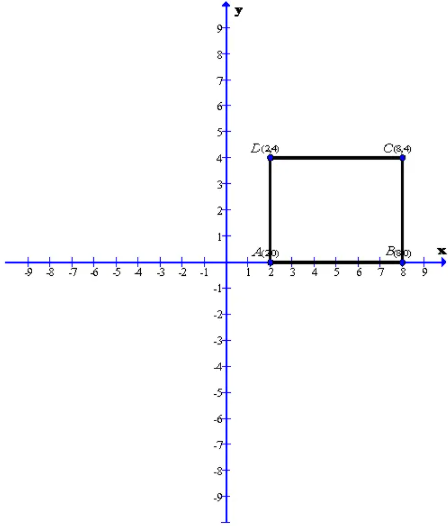After plotting the given points A(2,0), B(8,0) and C(8,4) on a graph paper; joining A with B and B with C. From the graph it is clear that the vertical distance between the points B(8,0) and C(8,4) is 4 units, therefore the vertical distance between the points A(2,0) and D must be 4 units. Now complete the rectangle ABCD
As is clear from the graph D(2,4)
(ii) A(4,2), B(-2,-2) and D(4,-2)

After plotting the given points A(4,2), B(-2,2) and D(4,-2) on a graph paper; joining A with B and A with D. From the graph it is clear that the vertical distance between the points A(4,2) and D(4,-2) is 4 units and the horizontal distance between the points A(4,2) and B(-2,2) is 6 units , therefore the vertical distance between the points B(-2,2)and C must be 4 units and the horizontal distance between the points B(-2,2) and C must be 6 units. Now complete the rectangle ABCD
As is clear from the graph C(-2,2)
(iii) A(-4, -6), C(6, 0) and D(-4, 0)

After plotting the given points A(-4, -6), C(6, 0) and D(-4, 0) on a graph paper ; joining D with A and D with C. From the graph it is clear that the vertical distance between the points D(-4, 0) and A(-4, -6) is 6 units and the horizontal distance between the points D(-4, 0) and C(6, 0) is 10 units, therefore the vertical distance between the points C(6, 0) and B must be 6 units and the horizontal distance between the points A(-4, -6) and B must be 10 units. Now complete the rectangle ABCD
As is clear from the graph B(6, -6)

(iv) B(10, 4), C(0, 4) and D(0, -2)After plotting the given points B(10, 4), C(0, 4) and D(0, -2) on a graph paper ; joining C with B and C with D. From the graph it is clear that the vertical distance between the points C(0, 4) and D(0, -2) is 6 units and the horizontal distance between the points C (0, 4) and B(10, 4) is 10 units, therefore the vertical distance between the points B(10, 4) and A must be 6 units and the horizontal distance between the points D(0, -2) and A must be 10 units. Now complete the rectangle ABCD.
As is clear from the graph A(10 , -2).

8. A (-2, 2), B(8, 2) and C(4, –4) are the vertices of a parallelogram ABCD. By plotting the given points on a graph paper; find the co-ordinates of the fourth vertex D.

Also, form the same graph, state the co-ordinates of the mid-points of the sides AB and CD

Given A(2, -2) , B(8, 2) and C(4 , -4) are the vertices of the parallelogram ABCDAfter plotting the given points A(2,-2), B(8,2) and C(4,-4) on a graph paper; joining B with C and B with A. Now complete the parallelogram ABCD.
As is clear from the graph D(-6,4)
Now from the graph we can find the mid points of the sides AB and CD.
Therefore the co-ordinates of the mid-point of AB is E(3,2) and the co-ordinates of the mid-point of CD is F(-1,-4).

9. A (-2, 4), C(4, 10) and D(-2, 10) are the vertices of a square ABCD. Use the graphical method to find the co-ordinates of the fourth vertex B. Also, find:
(i) The co-ordinates of the mid-point of BC;
(ii) The co-ordinates of the mid-point of CD and
(iii) The co-ordinates of the point of intersection of the diagonals of the square ABCD.

Given A(-2 , 4) , C(4, 10) and D(-2, 10) are the vertices of a square ABCD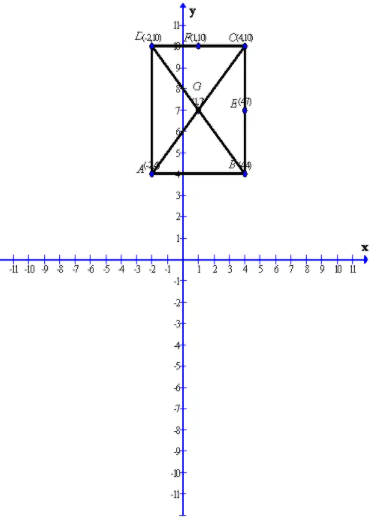After plotting the given points A(-2, 4) , C(4, 10) and D(-2, 10) on a graph paper; joining D with A and D with C. Now complete the square ABCD
As is clear from the graph B(4, 4)
Now from the graph we can find the mid points of the sides BC and CD and the co - ordinates of the diagonals of the square.
Therefore the co - ordinates of the mid - point of BC is E (4, 7) and the co- ordinates of the mid- point of CD is F(1, 10) and the co - ordinates of the diagonals of the square  is G(1, 7)

10. By plotting the following points on the same graph paper. Check whether they are col linear or not:
(i) (3, 5), (1, 1) and (0, -1)
(ii) (-2, -1), (-1, -4) and (-4, 1)

(ii) P (-2, -1), Q(-1, -4) and R(-4, 1) are non- collinear.

11. Plot point A(5, -7). From point A, draw AM perpendicular to the x-axis and AN perpendicular to the y-axis. Write the coordinates of points M and N

Given A (5, -7 )After plotting the given point A(5, -7) on a graph paper. Now let us draw a perpendicular AM form the point A(5, -7 )on the x - axis and a perpendicular AN from the point A(5, -7) on the y-axis.
As from the graph clearly we get the co - ordinates of the points M and N
Co-ordinate of the point M is (5, 0)
Co-ordinate of the point N is (0, -7)

12. In square ABCD; A = (3, 4), B = (-2, 4) and C = (-2, -1). By plotting these points on a graph paper, find the co-ordinates of vertex D. Also, find the area of the square

Given that in square ABCD ; A(3,4 ) , B(-2 , 4) and C(-2 , -1)

13. In rectangle OABC; point O is the origin, OA = 10 units along x-axis and AB = 8 units. Find the co-ordinates of vertices A, B and C

After plotting the given points A(3, 4) , B(-2, 4) and C(-2, -1 ) on a graph paper ; joining B with C and B with A. From the graph it is clear that the vertical distance between the points B(-2 , 4) and C(-2, -1) is 5 units and the horizontal distance between the points B(-2 , 4) and A(3, 4) is 5 units, therefore the vertical distance between the points A(3, 4) and D must be 5 units and the horizontal distance between the points C(-2, -1) and D must be 5 units. Now complete the square ABCD
As is clear from the graph  D(3, -1)
Now the area of the square ABCD is given by
area of ABCD = (side)2 = (5)2 = 25 units
Given that in rectangle OABC : point O is origin and OA = 10 units along x - axis therefore we get O(0, 0) and A(10, 0). Also it is given that AB = B units . Therefore we get B(10, 8) and C(0, 8)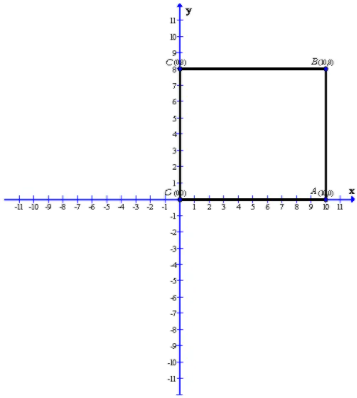After plotting the points O(0, 0) , A (10, 0) , B(10, 8) and C(0, 8) on a graph paper ; we get the above rectangle OABC and the required co -ordinates of the vertices are A(10, 0) , A(10, 0) , B(10, 8) and C(0, 8) on a graph paper ; we get the above rectangle OABC and the required co - ordinates of the vertices are A(10, 0) , B(10, 8) and C(0, 8)

### Exercise 26(B)

1. Draw the graph for each linear equation given below

(i) x = 3

(ii) x +3 =0

(iii) x -5 = 0

(iv) 2x-7 = 0

(v) y = 4

(vi) y + 6 = 0

(vii) y – 2 = 0

(viii) 3y + = 0

(ix) 2y – 5 = 0

(x) y = 0

(xi) x = 0

(i) Since x = 3, therefore the value of y can be taken as any real no.
First prepare a table as follows:

Thus the graph can be drawn as follows :(iii) First prepare a table as follows :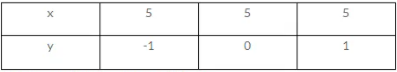Thus the graph can be drawn as follows:(iv) The equation can be written as :
x =7/2
First prepare a table as follows :Thus the graph can be drawn as follows :(v) First prepare a table as follows :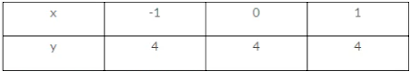Thus the graph can be drawn as follows: Thus the graph can be draw as follows: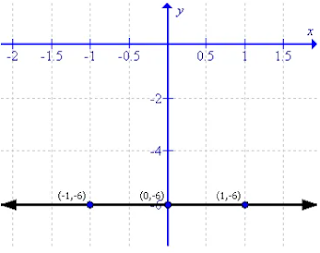(vii) First prepare a table as follows :Thus the graph can be drawn as follows :(viii) First prepare a table as follows :Thus the graph can be drawn as follows :(ix) First prepare a table as follows :Thus the graph can be drawn as follows :
(vii) First prepare a table as follows :
Thus the graph can be drawn as follows :

3. Draw the graph for each linear equation given below

(i) y = 2x + 3
(ii) y = 2x /3 -1
(iii) y = – x + 4
(iv) y = 4x – 5/2
(v) y = 3x/2 + 2/3
(vi) 2x – 3y = 4
(vii) (x-1)/3 - (y+2)/2 = 0

(viii) x - 3 = 2/5 (y+1)

(ix) x + 5y + 2 = 0

(i) First prepare a table as follows:

(ii) First prepare a table as follows :Thus the graph can be drawn as follows :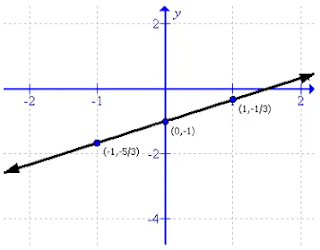(viii) The equation will become :
5x - 2y = 17
First prepare a table as follows :
Thus the graph can be drawn as follows :
(ix) First prepare a table as follows :
Thus the graph can be drawn as follows :

4. Draw the graph for each linear equation given below

(i) 3x + 2y =6
(ii) 2x – 5y =10
(iii) x/2 + 2y/3 = 5
(iv) (2x - 1)/3 - (y-2)/5 = 0

(i) To draw the graph of 3x + 2y = 6 follows the steps :
First prepare a table as below :

(ii) To draw the graph of 2x - 5y = 10 follows the steps :
First prepare a table as below :
From the graph it can verify that the line intersect x axis at (5, 0) and y at (0, -2).
(iii) To draw the graph of x/2 + 2y/3 = 3 follows the steps :
First prepare a table as below :
Now sketch the graph as shown :
From the graph it can verify that the line intersect x axis at (10, 0) and y at (0.7, 5).
(iv) To draw the graph of (2x - 1)/3 - (y-2)/5 = 0 follows the steps :
First prepare a table as below:
Now sketch the graph as shown :
From the graph it can verify that the line intersect x axis at (-1/10 , 0) and y at (0, 4.5).

5. For the linear equation, given above, draw the graph and then use the graph drawn (in the following case) to find the area of a triangle enclosed by the graph and the co-ordinates axes:

(i) 3x – (5 – y) = 7
(ii) 7 – 3 (1 – y) = – 5 + 2x.

(i) First draw the graph as follows :

This is an right triangle .
Thus the area of the triangle will be :
= (1/2) × base × altitude
= (1/2) × 4×12
= 24 sq. units
(ii) First draw the graph as follows :
This is a right triangle.
Thus the area of the triangle will be :
A = (1/2)×base ×altitude
= (1/2)× (9/2) ×3
= (27/4) = 6.75 sq. units

6. For the pair of linear equations given below, draw graphs and then state, whether the lines drawn are parallel or perpendicular to each other

(i) y = 3x – 1 , y = 3x + 2
(ii) y = x – 3 , y = – x + 5

(iii) 2x – 3y = 6 , x/2 + y/3 =1

(iv) 3x + 4y = 24, x/4 + y/3 = 1

(i) To draw the graph of y = 3x - 1 and y = 3x +2 follows the steps :
First prepare a table as below :Now sketch the graph as shown :

(ii) To draw the graph of  y = x -3 and y = -x + 5 follows the steps :
First prepare a table as below :
From the graph it can verify that the lines are perpendicular.
(iii) To draw the graph of 2x - 3y = 6 and x/2  + y/3 = 1 follows the steps :
First prepare a table as below :
(iv) To draw the graph of 3x +4y = 24 and x/4 + y/3 = 1 follows the steps :
First prepare a table as below :

7: On the same graph paper, plot the graph of y = x – 2, y = 2x + 1 and y = 4 from x= –4 to 3.

First prepare a table as follows :

8: On the same graph paper, plot the graphs of y = 2x – 1, y = 2x and y = 2x + 1 from x = – 2 to x = 4. Are the graphs (lines) drawn parallel to each other?

First prepare a table as follows :

9. The graph of 3x + 2y = 6 meets the x=axis at point P and the y-axis at point Q. Use the graphical method to find the co-ordinates of points P and Q.

To draw the graph of 3x + 2y = 6 follows the steps :
First prepare a table as below :From the graph it can verify that the line intersect x axis at (2,0) and y at (0,3), therefore the co ordinates of P(x - axis)  and Q(y - axis) are (2,0) and (0, 3) respectively.

10. Draw the graph of equation x + 2y – 3 = 0. From the graph, find:

(i) x1, the value of x, when y = 3
(ii) x2, the value of x, when y = – 2.

First prepare a table as follows :

(i) For y = 3 we have x = -3
(ii) For y = -2 we have x = 7

11. Draw the graph of the equation 3x – 4y = 12.
Use the graph drawn to find:
(i) y1, the value of y, when x = 4.
(ii) y2, the value of y, when x = 0.

First prepare a table as follows :

From the graph it can be verify that
If x = 4 the value of y = 0
If x = 0 the value of y = -3.

12. Draw the graph of equation x/4+y/5=1 Use the graph drawn to find:
(i) x1, the value of x, when y = 10
(ii) y1, the value of y, when x = 8.

First prepare a table as follows:

From the graph it can be verified that :
for y = 10, the value of x = -4 .
for x = 8 the value of  y = -5 .

13. Use the graphical method to show that the straight lines given by the equations x + y = 2, x – 2y = 5 and 3x+y=0 pass through the same point.

The equations can be written as follows :
y = 2 -x
⇒ y = (1/2)(x-5)
⇒ y = -x/3
First prepare a table as follows :

Thus the graph can be drawn as follows :

### Exercise 26(C)

1. In each of the following, find the inclination of line AB

(i)

(ii)
(iii)

The angle which a straight line makes with the positive direction of x-axis (measured in anticlockwise direction) is called inclination o the line.
The inclination of a line is usually denoted by θ
(i) The inclination is θ = 45°
(ii) The inclination is θ = 135°
(iii) The inclination is θ = 30°

2. Write the inclination of a line which is:
(i) Parallel to the x-axis
(ii) Perpendicular to the x-axis
(iii)Parallel to the y-axis.
(iv) Perpendicular to the y-axis.

(i) The inclination of a line parallel to x-axis is θ = 0°
(ii) The inclination of a line perpendicular to x-axis is θ = 90°
(iii) The inclination of a line parallel to y-axis is θ = 90°
(iv) The inclination of a line perpendicular to y-axis is θ = 0°

3. Write the slope of the line whose inclination is:

(i) 0
(ii) 30
(iii) 45

(iv) 60

If θ is the inclination of a line; the slope of the line is tanθ and is usually denoted by letter m.
(i) Here the inclination of a line is 0, then θ = 0°.
Therefore, the slope of the line is m = tan0° = 0

(ii) Here the inclination of a line is 30°, then θ = 30°.
Therefore the slope of the line is m = tanθ = 30° = 1/√3

(iii) Here the inclination of a line is 45° , then θ = 45°.
Therefore, the slope of the line is m = tan 45° = 1

(iv) Here the inclination of a line is 60°, then θ = 60°.
Therefore the slope of the line is m = tan 60° =√3

4. Find the inclination of the line whose slope is

(i) 0
(ii) 1
(iii) √3

(iv) 1/√3

If tan θ is the slope of a line; then inclination of the line is tan θ

(i) Here the slope of line is 0; then tan θ = 0

Now,

tan θ = 0

⇒ tan θ = tan 0°

⇒ θ = 0°

Therefore, the inclination of the given line is θ = 0°

(ii) Here the slope of line is 1 ; then tan θ = 1

Now,

tan θ = 1

⇒ tan θ = tan 45°

⇒ θ = 45°

Therefore, the inclination of the given line is θ = 45°

(iii) Here the slope of line is √3; then tan θ = √3

Now,

tan θ = √3

⇒ tan θ = tan 60°

⇒ θ = 60°

Therefore, the inclination of the given line is θ = 60°

(iv) Here the slope of line is 1/√3 ; then tan θ = 1/√3

Now,

tan θ = 1/√3

⇒ tan θ = tan 30°

⇒ θ = 30°

Therefore, the inclination of the given line is θ = 30°

5. Write the slope of the line which is

(i) Parallel to the x-axis

(ii) Perpendicular to the x-axis.

(iii) Parallel to the y-axis.

(iv) Perpendicular to the y-axis.

(i) For any line which is parallel to x - axis, the inclination if θ = 0°

Therefore, Slope (m) = tan θ = tan0° = 0

(ii) For any line which is perpendicular to x - axis, the inclination is θ = 90°

Therefore, Slope (m) = tan θ = tan 90° = ∞ (not defined)

(iii) For any line which is parallel to y - axis, the inclination is θ = 90°

Therefore, Slope (m) = tan θ = tan 90° =  ∞ (not defined)

(iv) For any line which is perpendicular to y - axis, the inclination is θ = 0°

Therefore, Slope (m)  = tan θ = tan 0° = 0

6. For the equation given below, find the slope and the y-intercept:

(i) x + 3y + 5 = 0

(ii) 3x – y – 8 = 0

(iii) 5x = 4y + 7

(iv) x= 5y – 4.

(v) y = 7x – 2

(vi) 3y = 7

(vii) 4y + 9 = 0

Equation of any straight line in the form y = mx + c, where slope = m (co-efficient of x) and y - intercept = c (constant term)

(i) x + 3y + 5 = 0

⇒ 3y = -x - 5

Therefore,
slope = co-efficient of x = -1/3
y - intercept = constant term = -5/3

(ii) 3x - y - 8 = 0
⇒ -y = -3x + 8
⇒ y = 3x + (-8)
Therefore,
slope = co - efficient of x = 3
y - intercept = constant term  = -8

(iii) 5x = 4 y + 7
⇒ 5x = 4y + 7
⇒ 4y = 5x - 7
Therefore,
slope = co-efficient of x = 5/4
y - intercept = constant term  = -7/4

(iv) x = 5y - 4
⇒ 5y = x + 4
Therefore,
slope = co-efficient of x = 1/5
y - intercept = constant term = 4/5

(v) y = 7x - 2
⇒ y = 7x + (-2)
Therefore,
slope = co - efficient of x = 7
y - intercept  = constant term  = - 2

(vi) 3y = 7
⇒ 3y= 0.x +7
Therefore,
slope = co-efficient of x = 0
y - intercept = constant term = 7/3

(vii) 4y + 9 = 0
4y = 0.x - 9
Therefore,
slope = co-efficient of x = 0
y - intercept = constant term = -9/4

7. Find the equation of the line whose:

(i) Slope = 2 and y-intercept = 3

(ii) Slope = 5 and y-intercept = – 8

(iii) slope = – 4 and y-intercept = 2

(iv) slope = – 3 and y-intercept = – 1

(v) slope = 0 and y-intercept = – 5

(vi) slope = 0 and y-intercept = 0

(i) Given,

Slope is 2, therefore m = 2

y - intercept is 3, therefore c = 3

Therefore,

y = mx + c

⇒ y = 2x + 3

Therefore, the equation of the required line is y = 2x + 3

(ii) Given

Slope is  5, therefore m = 5

Y - intercept is - 8, therefore c = -8

Therefore,

y = mx + c

⇒ y = 5x + (-8)

Therefore, the equation of the required line is y = 5x + (-8)

(iii) Given,

Slope is - 4, therefore m = -4

Y - intercept is  2, therefore c = 2

Therefore,

y = mx + c

⇒ y = -4x + 2

Therefore the equation of the required line is y = -4x + 2

(iv) Given

Slope is  -3 , therefore m = -3

Y - intercept is -1, therefore c = -1

Therefore,

y = mx + c

⇒ y = -3x - 1

Therefore the equation of the required line is y = -3x - 1

(v) Given

Slope is  0, therefore m = 0

Y - intercept is  - 5, therefore c = -5

Therefore,

y = mx + c

⇒ y = 0.x + (-5)

⇒ y = -5

Therefore the equation of the required line is y = -5

(vi) Given

Slope is 0, therefore m = 0

Y - intercept is 0 , therefore c = 0

Therefore,

y = mx +c

⇒ y =0.x + 0

⇒ y = 0

Therefore the equation of the required line is y = 0

8. Draw the line 3x + 4y = 12 on a graph paper. From the graph paper, read the y-intercept of the line

Given line is 3x + 4y = 12
The graph of the given line is shown below.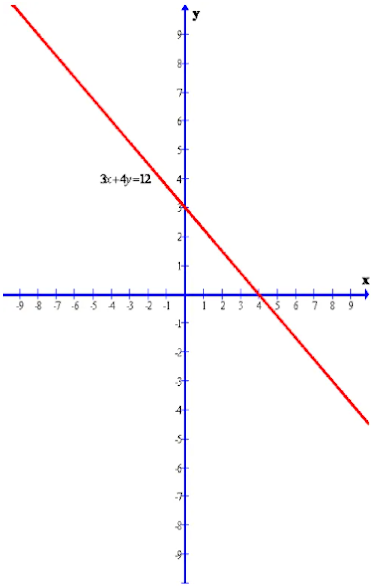Clearly from the graph we can find the y-intercept.
The required y-intercept is 3.

9.Draw the line 2x – 3y – 18 = 0 on a graph paper. From the graph paper, read the y-intercept of the line.

Given line is
2x – 3y – 18 = 0
The graph of the given line is shown below.

Clearly from the graph we can find the y-intercept.
The required y-intercept is -6

10. Draw the graph of the line x + y = 5. Use the graph paper drawn to find the inclination and the y-intercept of the line.

Given line is
x + y = 5
The graph of the given line is shown below.

From the given line x + y = 5, we get
x+ y = 5
⇒ y = -x +5
⇒ y = (-1).x + 5 ...(A)
Again, we know that equation of any straight line in the form y = mx +c , where m is the gradient and c is the intercept. Again we have if slope of a line is tanθ then inclination of the line is θ.
Now from the equation (A), we have
m = -1
⇒ tanθ = -1
⇒ tanθ = tan 135°
⇒ θ = 135°
and C = 5
Therefore, the required inclination is θ = 135° and Y - intercept is C = 5.